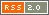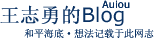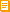订阅本博客如何订阅博客？王志勇 发表于 2019年09月18日 09:56

Ubuntu 16显示：/etc/php/7.4/fpm
Ubuntu 12显示：/etc/php5/fpm

php7.4-fpm和php5-fpm就是需要得到的服务名。

PHP官方公司的开发者，应该把Ubuntu/Debian系统的fpm服务名，都统一为php-fpm，就省很多麻烦

Nginx的PHP服务进程名：
Ubuntu 14下是php5-fpm
CentOS 6下是php-fpm
Ubuntu 16，自带的PHP软件源的进程是php7.0-fpm

PPA+PHP的fpm进程名：
PHP 7.4： php7.4-fpm
PHP 7.4： php7.3-fpm
PHP 5.6： php5.6-fpm

2019-09-18 10:37更新：

/etc/php/7.4/fpm
/etc/php/5.6/fpm

a1=`find /etc -name 'fpm'`; a2=(\${a1// /}); echo \${a2};

2019-09-18 10:53更新：更完美的解决、折腾方案

find /etc/php* -name 'fpm'

2019-09-18 11:14更新：

a1=`find /etc/php* -name 'fpm'`;
b1=(\${a1// /}); for a2 in \${b1[@]}; do echo \$a2; done;

2019-09-18 11:27更新：

a4='';
a3=`ps -ef | grep 'fpm'`;
a1=`find /etc/php* -name 'fpm'`;
b1=(\${a1// /});
a5=\${b1};
for a2 in \${b1[@]}; do if [[ \$a3 =~ \$a2 ]]; then a4=\$a2; fi; done;

if [ "\$a4"x = ''x ]; then a4=\$a5; fi;
…… (还少了一步把/etc/php/7.3/fpm转成php7.3-fpm，未写完)
if [ "\$a4"x != ''x ]; then service \$a4 restart; fi;

b1=(\${a1// /}); 这一行表示把空格变成数组，
if [ "\$a4"x = ''x ]; then a4=\$a5; fi; 这一行表示，如果ps -ef进程里没有fpm，则取find结果里的第一个fpm目录的名称，然后自动重启fpm。

2019-09-18 11:45更新：

a4='/etc/php/7.4/fpm';
a4=\${a4//\/etc\//};
a4=\${a4//\/fpm/};
a4=\${a4//\//}'-fpm';
echo \$a4;

php/7.4/fpm
php5/fpm

php/7.4
php5

php7.4
php5

php7.4-fpm
php5-fpm

if [ "\$a4"x != ''x ]; then a4=\${a4//\/etc\//}; a4=\${a4//\/fpm/}; a4=\${a4//\//}'-fpm';
service \$a4 restart;
fi;

a4='';
a3=`ps -ef | grep 'fpm'`;
a1=`find /etc/php* -name 'fpm'`;
b1=(\${a1// /});
a5=\${b1};
for a2 in \${b1[@]}; do if [[ \$a3 =~ \$a2 ]]; then a4=\$a2; fi; done;

if [ "\$a4"x = ''x ]; then a4=\$a5; fi;
if [ "\$a4"x != ''x ]; then a4=\${a4//\/etc\//}; a4=\${a4//\/fpm/}; a4=\${a4//\//}'-fpm';
service \$a4 restart;
fi;

0条评论：

 发表评论： 名字： (*必填) 博客： (可省)